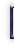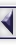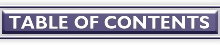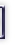# Numerical Workbenches

#### By Christoph Spiel

Some people think GNU/Linux is a good operating system, but has not enough applications to make it succeed in the market. Although this might be true for the desktop area, it is certainly wrong for numerical workbenches. In this field GNU/Linux users have many different (and excellent) choices -- in fact too many to introduce them all. Therefore, this series of articles introduces three outstanding applications:

GNU/Octave 2.1.34
http://www.che.wisc.edu/octave/
Scilab 2.6
http://www-rocq.inria.fr/scilab/
Tela 1.32
http://www.geo.fmi.fi/prog/tela.html

To find out about more numerical workbenches, check out http://sal.kachinatech.com/A/2/

## Introduction

What can these programs do? Isn't paper and pencil -- er -- a spreadsheet program enough?

The main application areas of numerical workbenches are:

• Preprocessing (yes -- meanwhile we need computers to talk to computers) and postprocessing data with the special case of ``gluing'' two numerical applications together.
• Numerical optimizations (both, linear and non-linear),
• Standalone simulations,
• Data visualization,
• Sophisticated ``pocket'' calculators,
• Rapid prototyping of specialized numerical applications, which finally will be implemented in, say, C++ or Fortran-90.

However, because all of them provide complete programming languages to the user and, moreover, are designed to be extended, the number of numerical problems they can solve is almost limitless.

### Numerical Mathematics

Now, what the heck is numerical math anyhow? Numerical Mathematics is the branch of math that develops, analyzes, and applies methods to compute with finite precision numbers. Computer hardware, for example, uses numerical math.

Why do computers work with finite precision numbers? Why has nobody developed a scheme that allows for the storage of exact numbers?

1. Solving problems with finite precision numbers is faster -- much, much faster. Take for example the sum of all square roots from one to one million. On my computer, doing the exact computation with MuPAD-1.4.2 available at http://math-www.uni-paderborn.de/MuPAD/index.html
```    time(sum(sqrt(i), i = 1..10^6));
```

takes about 40 seconds, whereas getting the approximate result with Tela-1.32

```    tic(); sum(sqrt(1:10^6)); toc();
```

takes 0.31 seconds, that is, the answer in finite precision is returned over 100 times faster! Put another way, we can crunch hundred times more data with finite precision numbers in the same time slice.

2. When using good algorithms -- the ones suggested by numerical mathematicians -- and being careful one can get surprisingly precise answers even with finite precision numbers.

3. Admit it, most of the time users do not need exact results! A good approximation -- with ``sufficiently many correct digits'' -- will do.

### Article Organization

In this article series, we point out the similarities among the three applications that we are going to discuss. We will use GNU/Octave in most of the examples. Where there are important differences you should be aware of, we have put a Differences paragraph at the end of the section.

Technical details for the terminally curious have been put in Details sections.

## Getting In and Out

To give you a hands-on experience, let us start each of the applications, request help on a function, and then quit.

GNU/Octave
```    cspiel@hydra:~/articles/numerical-workbenches \$ octave
GNU Octave, version 2.1.34 (i686-pc-linux-gnu).
Copyright (C) 1996, 1997, 1998, 1999, 2000, 2001 John W. Eaton.
This is free software with ABSOLUTELY NO WARRANTY.
For details, type `warranty'.
```
```    *** This is a development version of Octave.  Development releases
*** are provided for people who want to help test, debug, and improve
*** Octave.
***
*** If you want a stable, well-tested version of Octave, you should be
*** using one of the stable releases (when this development release
*** was made, the latest stable version was 2.0.16).
```
```    octave:1> help diag
diag is a built-in function
```
```     - Built-in Function:  diag (V, K)
Return a diagonal matrix with vector V on diagonal K.  The second
argument is optional.  If it is positive, the vector is placed on
the K-th super-diagonal.  If it is negative, it is placed on the
-K-th sub-diagonal.  The default value of K is 0, and the vector
is placed on the main diagonal.  For example,
```
```              diag ([1, 2, 3], 1)
=>  0  1  0  0
0  0  2  0
0  0  0  3
0  0  0  0
```
```    octave:2> quit
cspiel@hydra:~/articles/numerical-workbenches \$
```

Alternatively use `exit` or press C-d to quit GNU/Octave.

GNU/Octave offers the user function-name completion, this is, when only part of a function's name is entered and the user hits Tab, the partial name is completed as much as possible. A second Tab displays the list of remaining choices.

Scilab
After starting Scilab thus:
```    cspiel@hydra:~/articles/numerical-workbenches \$ scilab
```

we get a new X-window in which the Scilab interpreter runs. Asking for help opens an xless(1x) window. (Both these links are screenshots, so click on them.)

To exit Scilab, enter `quit` or `exit`.

Scilab can also be launched in non-window mode by passing the ``` -nw``` option to it:

```    cspiel@hydra:~/articles/numerical-workbenches \$ scilab -nw
===========
S c i l a b
===========
```
```                              scilab-2.6
```
```    Startup execution:
```
```    -->help diag
```

The help system then uses the text output, too.

Tela
Tela's banner is quite terse, nonetheless, the help system is as comprehensive as necessary. Note that Tela offers function name completion as GNU/Octave does.
```    cspiel@hydra:~/articles/numerical-workbenches \$ tela
This tela is a tensor language, Version 1.32.
Type  ?help  for help.
->TAB completion works; try docview() and source("demo")
>help diag
diag(V, K) (V is a vector) returns a square diagonal matrix, with
vector V on the main diagonal (K == 0, default), the K-th super
diagonal (K > 0) or the K-th sub-diagonal (K < 0).
```
```       diag(M, K) (M is a matrix) returns the main diagonal (K == 0,
default), the K-th super diagonal (K > 0), or the K-th sub-diagonal
(K < 0) of M as a vector.  M need not necessarily be square.
```
```    >quit()
63 instructions, 0 arithmetic ops.
0.0063 MIPS, 0 MFLOPS.
cspiel@hydra:~/articles/numerical-workbenches \$
```

Tela can also be exited by pressing C-d.

## Better Than a Pocket Calculator!

Now that we know how to start and exit the programs, let us look at them in action.

### Simple Expressions

We want to see:

1. Whether we can write mathematical expressions the way we are used to from school. Ugh!
`1 + 2 * 3 ^ 4` should be treated as `1 + (2 * (3 ^ 4))`, yielding `163`. It should not be treated as `((1 + 2) * 3) ^ 4`, which equals `6561`,
2. How many bits are necessary to store `10^6`, and
3. How steep is our driveway? (measured in degrees) Our garage is 7 meters away from the street and half a meter above it.

Here we go.

```    cspiel@orion:~/articles/numerics \$ octave
```

All three programs are console-based. That is, the user gets a prompt whenever the application is ready to accept input. We enter our first question as we write it on paper. Hitting return terminates the line, the program evaluates it, and returns the result in variable `ans` (more on variables later).

```    octave:1> 1 + 2 * 3 ^ 4
ans = 163
```

Aha, obviously GNU/Octave knows elementary-school math!

Our second question requires the logarithm function `log`, which returns the natural logarithm of its argument; this is, the logarithm to base e.

```    octave:2> log(10^6) / log(2)
ans = 19.932
```

We conclude that 1,000,000 needs 20 bits to be stored.

Finally, how steep is our driveway? What we need here is an angular function, namely the arctangent, written as `atan(argument)`.

```    octave:3> atan(0.50 / 7.0)
ans = 0.071307
```

Hmm, ain't that a bit too flat? Digging out the wisdom of long forgotten math classes, we remember that the arctangent of 1 is 45 degrees. Let us check this!

```    octave:4> atan(1)
ans = 0.78540
```

Ouch, off by a factor of 57! Do we have to throw the program away? Wait -- 57 equals almost 180 over pi. This means GNU/Octave has returned the result in radians, not in degrees. All angular functions work in units of radians, this is, an angle of 360 degrees is equivalent 2 pi radians.

We try again, supplying the correct conversion factor:

```    octave:5> atan(0.50 / 7.0) * 360/(2 * 3.14)
ans = 4.0856
```

Approximately 4 degrees, that looks good. Our garage certainly won't get flooded in the next deluge.

Details

• Numbers are either real-valued or complex-valued. Elementary operations ``+'', ``-'', ``*'', and ``/'', as well as exponentiation ``^'' work as expected on reals and complex numbers.
• The commonly used basic functions are the absolute value ``` abs(arg)```, the sign-function `sign(arg)`, and the square root `sqrt(arg)`.
• Two logarithm functions are supplied, one to the base of e: `log(arg)`, and to the base of 10: `log10(arg)`. The exponential `exp(arg)` is the inverse of ``` log(arg)```.
• All workbenches offer a wealth of angular and hyperbolic functions: ``` sin(arg)```, `cos(arg)`, `tan(arg)`, ``` sec(arg)```, `csc(arg)`; `asin(arg)`, ``` acos(arg)```, `atan(arg)`, `acsc(arg)`; ``` sinh(arg)```, `cosh(arg)`, `tanh(arg)`, ``` sech(arg)```, `csch(arg)` `asinh(arg)`, ``` acosh(arg)```, `atanh(arg)`, `acsch(arg)`.

Differences

• Tela does not know the convenience variable `ans`.
• In GNU/Octave and Tela imaginary literal numbers are written by appending ``i'', ``j'', ``I'', or ``J'' to a number. For example `1i`, ``` -8.99I```, `324J`. Scilab defines a special constant for the imaginary unit `sqrt(-1)`, which is written <%i>. Therefore, Scilab's imaginary literals look like products: `-8.99*%i`, ``` %i*324```.

### Variables

In the last section we have not gained much in comparison with a pocket calculator, have we? The first feature where our programs beat pocket calculators and spread-sheets are names that we can give parameters or results; these are called variables.

Assume our better half wants us to build a garden in the yard, but we want to watch basketball. Therefore we quickly need a hard figure that proves we don't have enough compost for the desired size. Ha -- brilliant idea!From our little plan we take the following lengths in feet:

```    houseside_length = 10
creekside_length = 6
width = 2
```

Our better half also said the layer of new soil ought to be at least five inches, so

```    height = 5 / 12
```

GNU/Octave to the rescue!

```    octave:1> houseside_length = 10
houseside_length = 10
octave:2> creekside_length = 6
creekside_length = 6
octave:3> width = 2
width = 2
octave:4> height = 5 / 12
height = 0.41667
octave:5> volume = (houseside_length + creekside_length) * width * height
volume = 13.333
```

The compost box is 6' x 4' and currently holds eight inches of usable compost.

```    octave:6> box_width = 6
box_wight = 6
octave:7> box_depth = 4
box_depth = 4
octave:8> compost_height = 8/12
compost_height = 0.66667
octave:9> compost_volume = box_width * box_depth * compost_height
compost_volume = 16
```

Oh no, we have just dug our own grave. We have got enough compost! What about taping the match on the VCR?

Details

• Variables spring into existence when they are first assigned to.
• It is not an error to assign a value of different type to an existing variable. (Currently, we only know of one type, but more types are waiting for us)
• Using a undefined variable on the right-hand side of an assignment causes an error.

## Structured Data

Until now we have not exploited where computers are really good at: repetitive work.

### Vectors

Say we got a long receipt from the grocery store. [Your ad here!] How can we get the VAT in Dollars on each item given the gross amount and the VAT rate in percent? The formula

```            vat_percent / 100
vat = --------------------- * gross_amount
1 + vat_percent / 100
```

is trivial, but we want to save us repeated typing.

The list of all gross amounts in the receipt forms what numerical programs call a vector. Vectors are built from values by enclosing these values in square brackets and separating them with commas like this:

```    octave:1> gross = [1.49, 4.98, 0.79, 5.49, 0.96, 0.96, 0.96, 0.96]
gross =
```
```      1.49000  4.98000  0.79000  5.49000  0.96000  0.96000  0.96000  0.96000
```

The vector is built from left to right using our supplied numbers in the same order that we enter them.

Wouldn't it be wonderful if we simply wrote: ```gross * (vat_percent/100) / (1 + vat_percent/100)``` and get the VAT of each item? It really is that simple.

```    octave:2> vat_percent = 7
vat_percent = 7
octave:3> a = (vat_percent/100) / (1 + vat_percent/100)
a = 0.065421
octave:4> vat = a * gross
vat =
```
```      0.097477  0.325794  0.051682  0.359159  0.062804  0.062804  0.062804  0.062804
```

Wow -- it works! For the first time we have really gained convenience and expressiveness: a single multiplication sign performs eight multiplications in a row.

What has happened? `vat_percent` is a single value, which is called scalar in numerics to distinguish it from vectors. Well, if `vat_percent` is a scalar, then `vat_percent/100`, `1 + vat_percent/100`, and `a` are scalars, too. Finally, scalar `a` must be multiplied with vector ``` gross```. What we wanted and what happened was that `a` was multiplied in turn with every element of `gross`. This holds for every operator, not only multiplication! In general

vector  op  scalar

and

scalar  op  vector

apply scalar to every element in vector according to operation op. In our example, this is as if we had written the following

```    vat(1) = a * gross(1)
vat(2) = a * gross(2)
...
vat(8) = a * gross(8)
```

where we have introduced a new piece of syntax: vector indexing. Each element (a scalar) of a vector can be accessed by its index, which is the number of it's place in the vector. The index is written in parenthesis after the vector. For example, to get the second element in ``` gross```, we write

```    octave:5> gross(2)
ans = 4.9800
```

Elements in vectors can be assigned to with the same syntax. Just place the indexed vector to the left of the assignment sign, for example, ```gross(2) = 5.12```.

What else can be thought of as a vector of numbers besides our receipt? Any series of values! Most of the time the values will be related, like the temperature measured each day at 8am, the diameters of a batch of metal rods, the velocities of all westbound traffic across Buffalo Street at the corner of West Clinton Street on Wednesday April 18, 2001. As we are living in the Digital Age, many more series of data fit the notion of a vector: every piece of music on a CD is a vector of sound amplitudes and the indices mark discrete moments in time.

Details

• Mathematically speaking what we call vectors here are tuples.
• Most built-in functions, for example `sin`, can be used on vectors.
```    v = [0.12, 0.89, 0.78, 0.10]
sin(v)
```

or

```    sin([0.12, 0.89, 0.78, 0.10])
```
• Vectors do not have to be built from scalars; more than one vector can be catenated with the same syntax. Furthermore, the elements used in the definition of the vector do not have to be literal numbers, but can be arbitrary expressions, which again yield scalars or vectors.

Differences

• Tela uses a different syntax for vector construction, which resembles Scheme or Smalltalk: The vector is surrounded by parentheses which are preceded by a sharp sign; for example: ```gross = #(1.49, 4.98, ..., 0.96)```.
• Tela uses a different syntax for vector indexing, which resembles Pascal. The index is enclosed in square brackets; for example: `gross`. Warning for C programmers: Though the square brackets look like C, the lowest index always is 1.

## Next Month

• Matrices
• User defined functions
• Flow control statements
• Input and output

####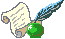Christoph Spiel

Chris runs an Open Source Software consulting company in Upper Bavaria/Germany. Despite being trained as a physicist -- he holds a PhD in physics from Munich University of Technology -- his main interests revolve around numerics, heterogenous programming environments, and software engineering. He can be reached at cspiel@hammersmith-consulting.com.

##### Copyright © 2001, Christoph Spiel. Copying license http://www.linuxgazette.net/copying.html Published in Issue 69 of Linux Gazette, August 2001